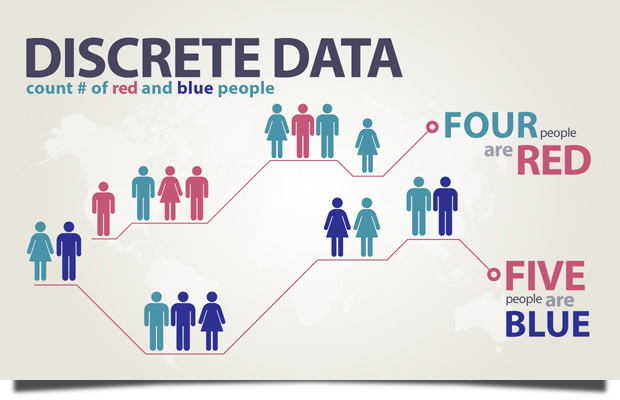# Discrete Data

Discrete Data results when the number of possible values is either a finite number or a countable number.

- Triola (2011).

# ExampleThis infographic looks at a population and counts how many are red and blue. Since these values are countable, it makes the data discrete.

Data

Continuous Data